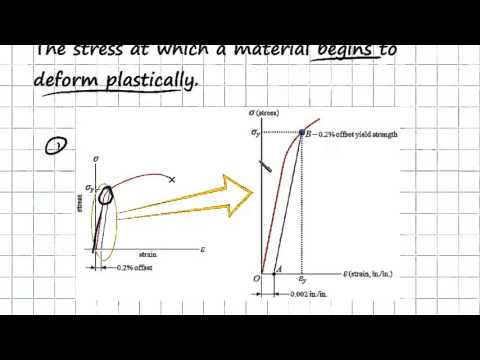### Main Difference

Before differentiating between yield strength and tensile strength one should be well aware with the terms like stress and strain as the main concepts of both these terms underlay both these strengths. The force applied can deform the objects, stress and strain are interrelated and have a strong connection with the deforming forces. Stress is the measure of deforming force per unit area of the body, whereas strain is the relative change in the body’s length due to the deforming forces. Stress has the similar unit as of pressure, Pascal (Pa), whereas in strain where its all about change in length, it is denoted as the percentage wise change in length of the body due to deforming force. It should be kept mentioned that more the stress on the object means more the strain. Following these concepts, yield stress is the minimum stress under which the object that leads to a permanent deformation, whereas tensile strength is the maximum stress which an object can bear before breaking or collapsing. The deformation we are talking here is the strain and is being caused by the stress on the surface of the object. Elasticity is another related terms as it is the ability of the object to bear the stress and to get the object back in the original condition.

### What is Yield Strength?

When the object is placed and no external force or stress is applied on it, no change comes in it. Actually the stress applied brings the deformation in the object, whereas the other forces can change its motion from static to kinetic or vice versa. The stress applied doesn’t brings the change rapidly, it depends upon the nature of the object and the stress being applied on it. At first one the stress is being applied it seems that the object might have deformed but it comes back to the actual shape or size, that is due to the elasticity of that object. Although at one point, when the stress is being applied the object deforms permanently and doesn’t comes back to the original position. The minimum stress under which the object leads to a permanent deformation is the yield strength.

### Tensile Strength?

The concept of tensile strength also revolves around stress and strain and it is the point that comes after the yield strength. When the stress is applied on the object and it deforms permanently, it is the yield strength, although when the stress is even being applied after the deformation of the object, a point comes when the object breaks or collapse. The tensile strength is the maximum stress which an object can bear before breaking or collapsing. For instance, a rubber band has one of the maximum tensile strengths as it has more elasticity, which means it can bear more stress before collapsing. When stress is applied on the rubber band it deforms or stretches so long, but finally the time comes when it gets broke.

### Yield Strength vs. Tensile Strength

• Stress is the measure of deforming force per unit area of the body, whereas strain is the relative change in the body’s length due to the deforming forces.
• Yield stress is the minimum stress under which the object that leads to a permanent deformation, whereas tensile strength is the maximum stress which an object can bear before breaking or collapsing.
• Elasticity is another related terms as it is the ability of the object to bear the stress and to get the object back in the original condition.